# 计算统计学与数据科学 06：线性模型选择与正则化

## 墨尔本大学 MAST90083 课程笔记

Posted by YEY on September 7, 2020

# Lecture 06 线性模型选择与正则化

• Gareth, J., Daniela, W., Trevor, H., & Robert, T. (2013). An intruduction to statistical learning: with applications in R. Spinger.
• Hastie, T., Tibshirani, R., & Friedman, J. (2009). The elements of statistical learning: data mining, inference, and prediction. Spinger Science & Business Media.

## 1. 引言

$Y=\beta_0 + \beta_1 X_1 +\cdots + \beta_p X_p + \epsilon$

• 预测准确率：若响应变量和预测变量的真实关系近似线性，则最小二乘估计的偏差较低。若 $n\gg p$，即观测个数 $n$ 远大于变量个数 $p$，则到最小二乘估计的方差通常较低，从而在测试样本集上有较好表现。然而，在不满足 $n$ 远大于 $p$ 的情况下，最小二乘拟合可能会发生较大变化，发生过拟合，从而使模型在测试样本集上表现较差。此外，若 $p > n$，最小二乘法得到的系数估计结果不唯一：此时方差为 无穷大 (infinite)，这种情况下最小二乘法会失效。通过 限制 (constraining)收缩 (shrinking) 待估计系数，以牺牲偏差为代价，显著减小估计量方差。这种方法可以显著提高模型在测试样本集上的预测准确率。

• 模型可解释性：通过删除不相关的特征，即通过将相应的系数估计设置为零，我们可以获得更易于解释的模型。 我们将介绍一些自动执行特征选择的方法

• 子集选择 (Subset selection)：该方法从 $p$ 个预测变量中挑选出与响应变量相关的变量，构建一个变量子集，再对这个缩减后的变量集合应用最小二乘法拟合模型。

• 收缩 (Shrinkage)：该方法基于全部 $p$ 个变量进行模型拟合。但是，与最小二乘估计相比，该方法的估计系数可以缩减为 $0$。这种收缩，也称 正则化 (regularization)，具有减少方差的效果。根据收缩的类型，某些系数估计可以正好为 $0$。因此，收缩法也可以用于变量选择。

• 降维 (Dimension reduction)：此方法将 $p$ 个原始预测变量投影到一个 $M$-维子空间中，其中 $M< p$。这是通过计算原始变量的 $M$ 个不同的 线性组合 (linear combination) 或者 投影 (projections) 实现的。然后，我们将这 $M$ 个投影作为新的预测变量，用最小二乘法拟合线性回归模型。

## 2. 子集选择

### 2.1 最优子集选择

1. 令 $\mathcal M_0$ 表示 零模型 (null model)，即不包含任何预测变量的模型。该模型只是简单预测各观测的样本均值。
2. 对于 $k=1,2,\dots,p$：
(a) 拟合正好包含 $k$ 个预测变量的所有 ${p \choose k}$ 个模型。
(b) 从这 ${p \choose k}$ 个模型中选择具有最小 $\mathrm{RSS}$ 或者最大 $R^2$ 的模型作为最优模型，记为 $\mathcal M_k$。
3. 根据 $\mathrm{CV}$ 预测误差、$C_p\, (\mathrm{AIC})$、$\mathrm{BIC}$ 或者调整 $R^2$，从 $\mathcal M_0,\dots, \mathcal M_p$ 中选出一个最优模型。

#### 例子：信用卡数据集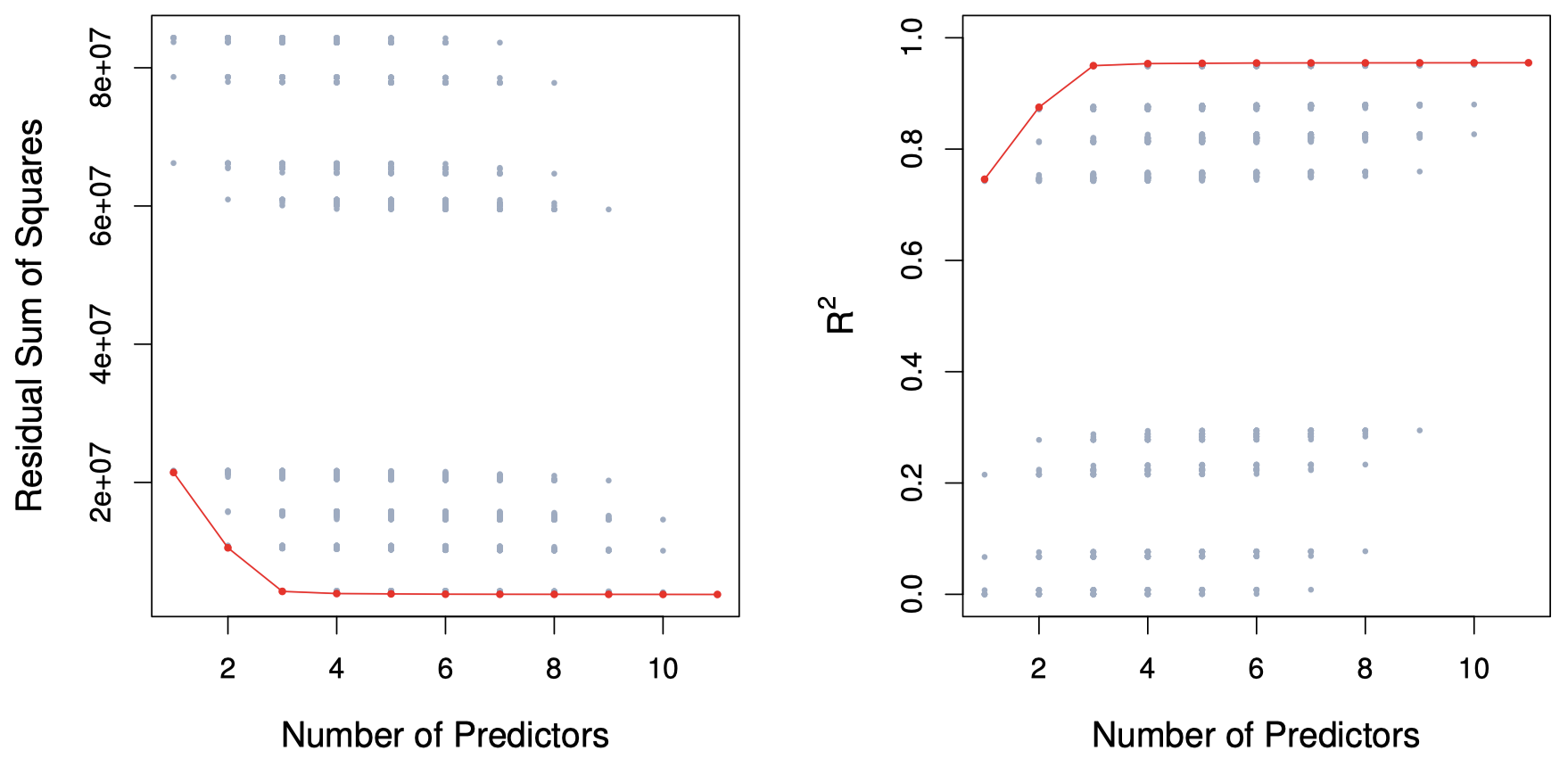### 2.2 逐步选择

• 由于 运算效率 的限制，最优子集选择方法不再适用。

• 另外，还存在一些统计学上的问题：随着搜索空间的增大，通过最优子集法找到的模型虽然在训练数据上有较好表现，但对新数据并不具备良好的预测能力。从一个巨大搜索空间中得到的模型通常会存在 过拟合系数估计方差高 的问题。

#### 前向逐步选择

1. 令 $\mathcal M_0$ 表示 零模型 (null model)，即不包含任何预测变量的模型。
2. 对于 $k=0,1,2,\dots,p-1$：
(a) 考虑所有 $p-k$ 个模型，其中每个模型都在模型 $\mathcal M_k$ 的基础上增加一个变量。
(b) 从这 $p-k$ 个模型中选择具有最小 $\mathrm{RSS}$ 或者最大 $R^2$ 的模型作为最优模型，记为 $\mathcal M_{k+1}$。
3. 根据 $\mathrm{CV}$ 预测误差、$C_p\, (\mathrm{AIC})$、$\mathrm{BIC}$ 或者调整 $R^2$，从 $\mathcal M_0,\dots, \mathcal M_p$ 中选出一个最优模型。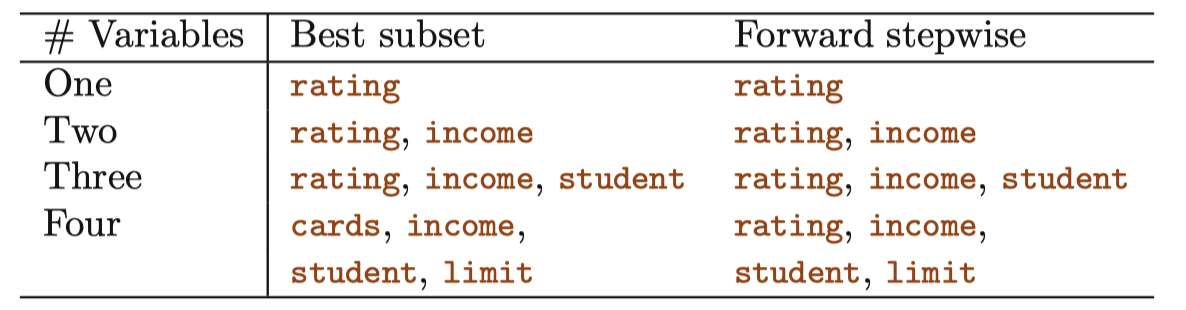#### 后向逐步选择

1. 令 $\mathcal M_p$ 表示 全模型 (full model)，即包含全部 $p$ 个预测变量的模型。
2. 对于 $k=p,p-1,\dots,1$：
(a) 考虑所有 $k$ 个模型，其中每个模型都在模型 $\mathcal M_k$ 的基础上减少一个变量，即每个模型都包含 $k-1$ 个变量。
(b) 从这 $k$ 个模型中选择具有最小 $\mathrm{RSS}$ 或者最大 $R^2$ 的模型作为最优模型，记为 $\mathcal M_{k-1}$。
3. 根据 $\mathrm{CV}$ 预测误差、$C_p\, (\mathrm{AIC})$、$\mathrm{BIC}$ 或者调整 $R^2$，从 $\mathcal M_0,\dots, \mathcal M_p$ 中选出一个最优模型。

### 2.3 选择最优模型

1. 根据过拟合导致的偏差对训练误差进行 调整，从而间接地估计测试误差。
2. 通过验证集方法或交叉验证方法，直接 估计测试误差。

#### $C_p$、$\mathrm{AIC}$、$\mathrm{BIC}$ 和调整 $R^2$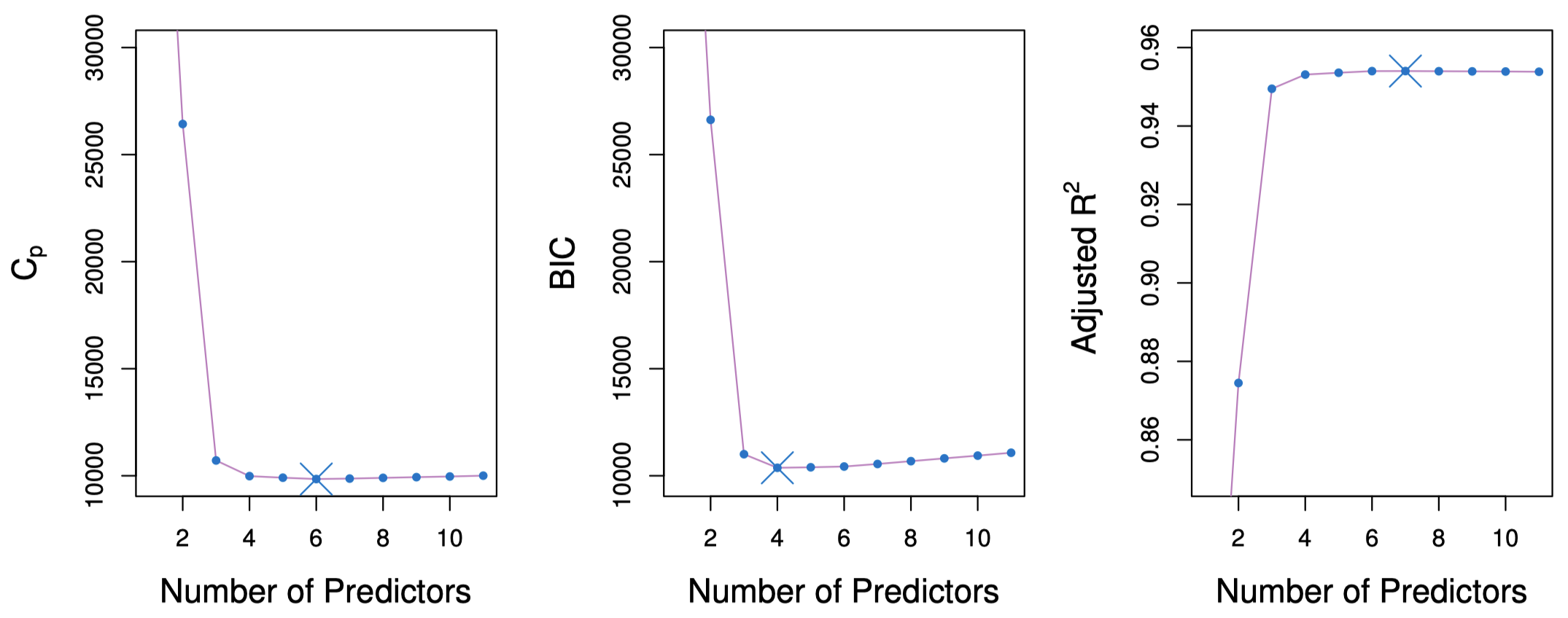##### Mallow’s $C_p$

$C_p= \dfrac{1}{n}(\mathrm{RSS} + 2d \hat \sigma^2)$

##### $\mathrm{AIC}$ 准则

$\mathrm{AIC}$ 准则适用于许多使用最大似然法进行拟合的模型：

$\mathrm{AIC} = -2 \log L + 2\cdot d$

##### $\mathrm{BIC}$ 准则

$\mathrm{BIC}$ 是从贝叶斯观点中衍生出来的，然而最终也和 $C_p$ (以及 $\mathrm{AIC}$) 非常相似。对于包含 $d$ 个变量的最小二乘模型，$\mathrm{BIC}$ 通常由下式给出：

$\mathrm{BIC} = \dfrac{1}{n}(\mathrm{RSS} + \log (n)d \hat \sigma^2)$

##### 调整 $R^2$

$\text{Adjusted } R^2=1- \dfrac{\mathrm{RSS}/(n-d-1)}{\mathrm{TSS}/(n-1)}$

#### 验证集法与交叉验证法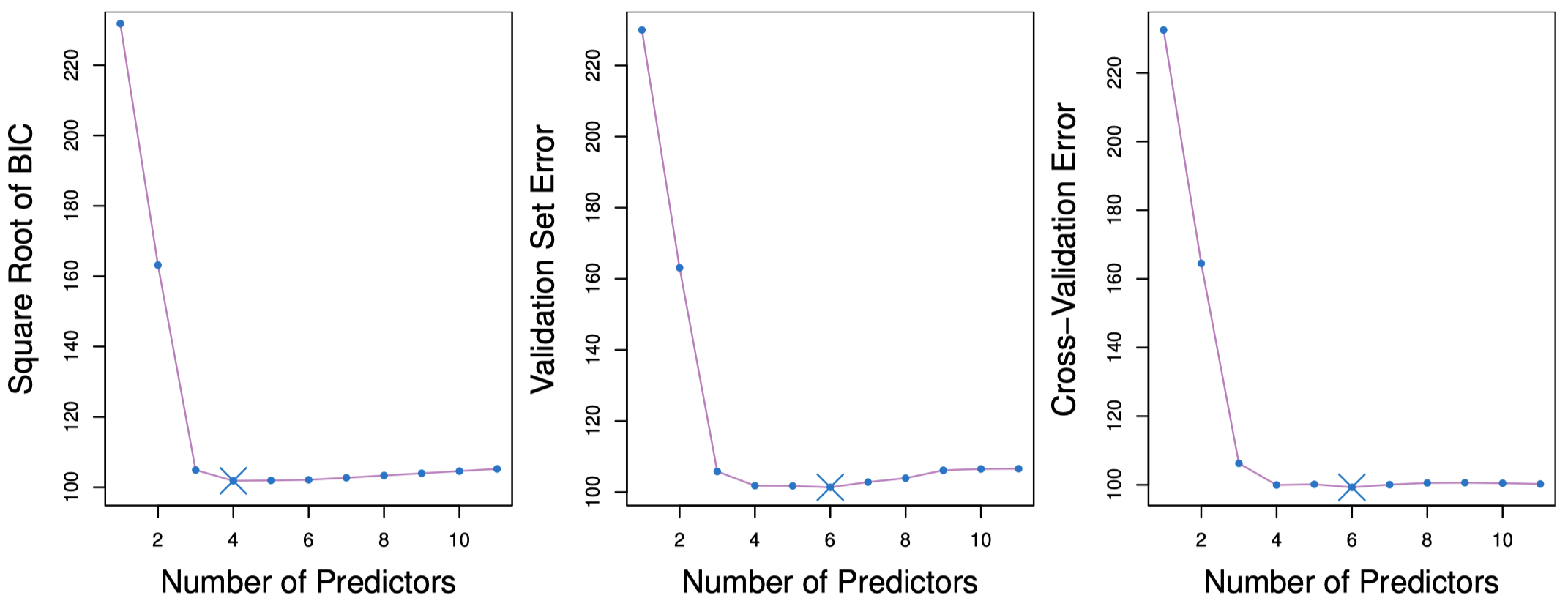## 3. 收缩方法

### 3.1 岭回归

$\mathrm{RSS}=\sum_{i=1}^{n}\left(y_i - \beta_0 - \sum_{j=1}^{p}\beta_j x_{ij}\right)^2$

$\sum_{i=1}^{n}\left(y_i - \beta_0 - \sum_{j=1}^{p}\beta_j x_{ij}\right)^2 + \lambda \sum_{j=1}^{p}\beta_j^2 = \mathrm{RSS} + \lambda \sum_{j=1}^{p}\beta_j^2$

#### 例子：信用卡数据集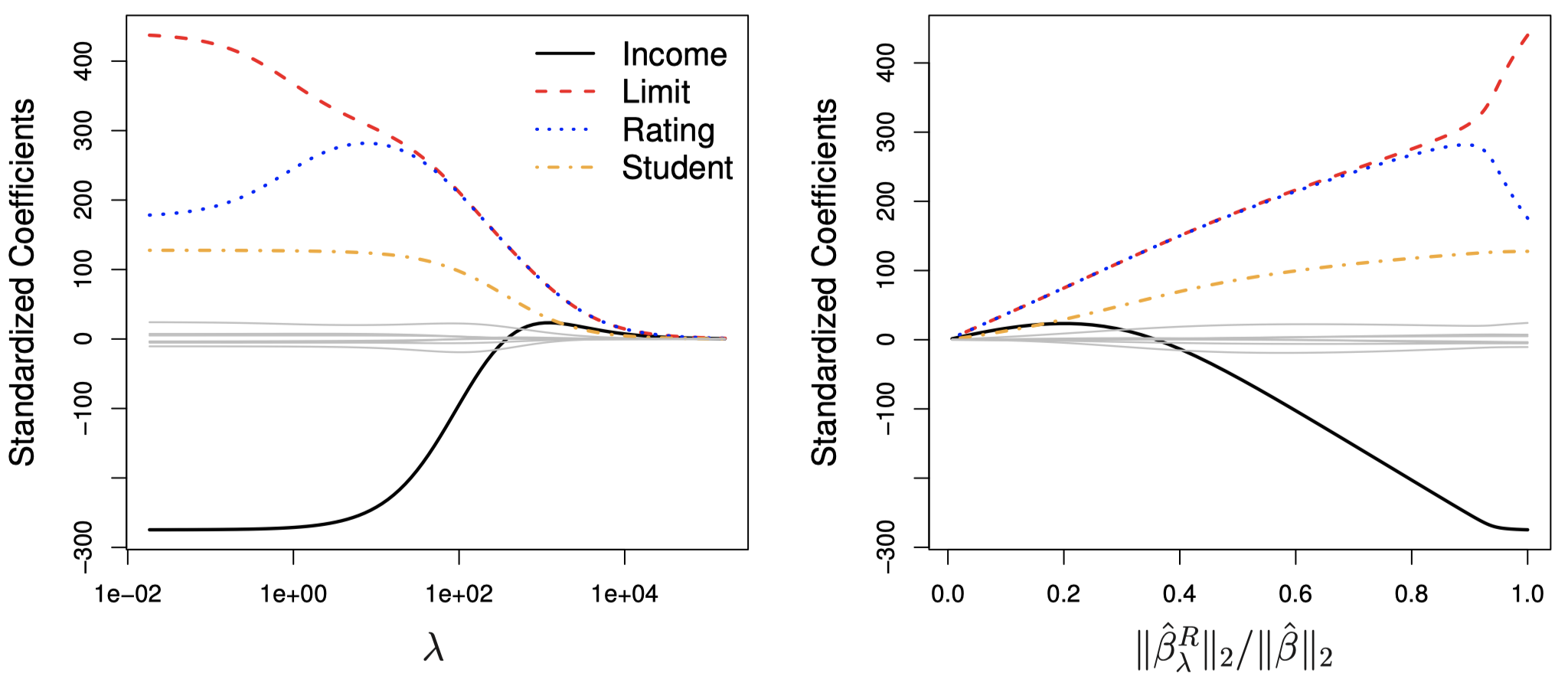#### 变量缩放

$\tilde x_{ij} = \dfrac{x_{ij}}{\sqrt{\dfrac{1}{n}\sum_{i=1}^{n}(x_{ij}- \overline x_j)^2}}$

#### 为什么岭回归要比最小二乘回归效果更好？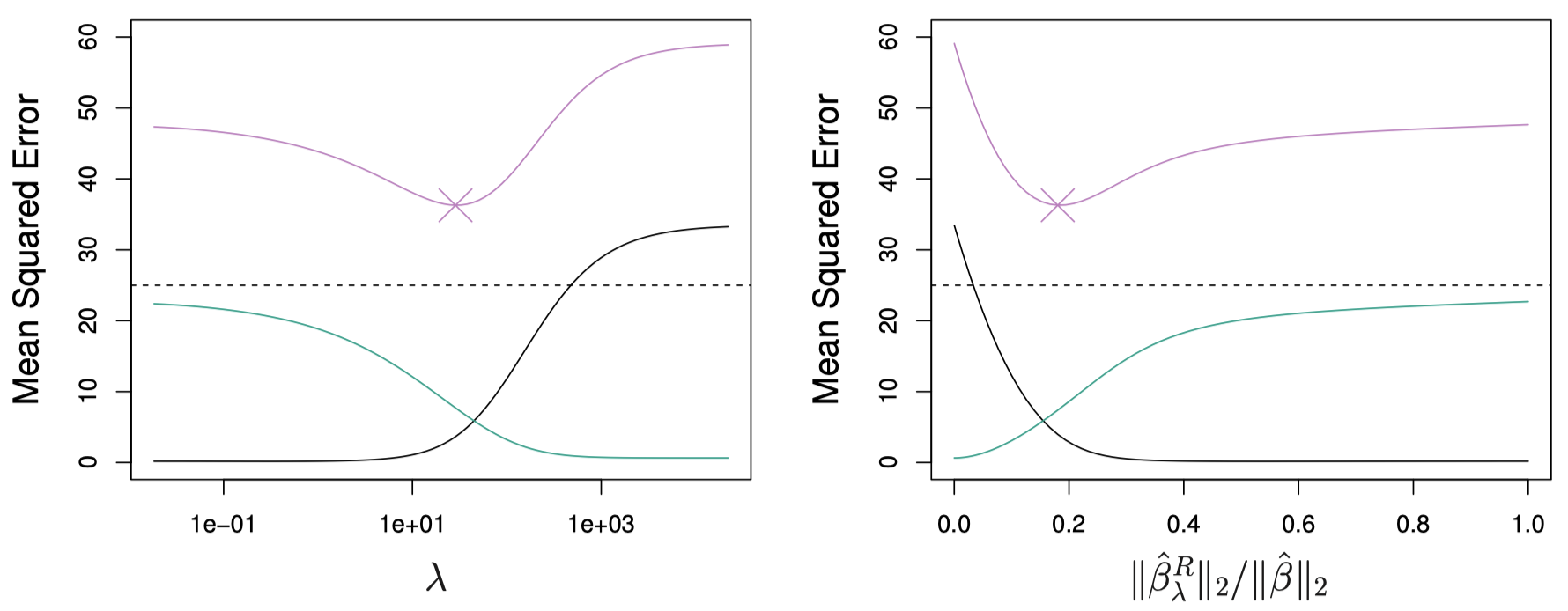### 3.2 Lasso

Lasso 是一种最近非常流行的用于克服岭回归缺点的方法。Lasso 的估计系数 $\hat \beta_{\lambda}^L$ 可以通过最小化下式得到：

$\sum_{i=1}^{n}\left(y_i - \beta_0 - \sum_{j=1}^{p}\beta_j x_{ij}\right)^2 + \lambda \sum_{j=1}^{p} |\beta_j | = \mathrm{RSS} + \lambda \sum_{j=1}^{p} |\beta_j |$

#### 例子：信用卡数据集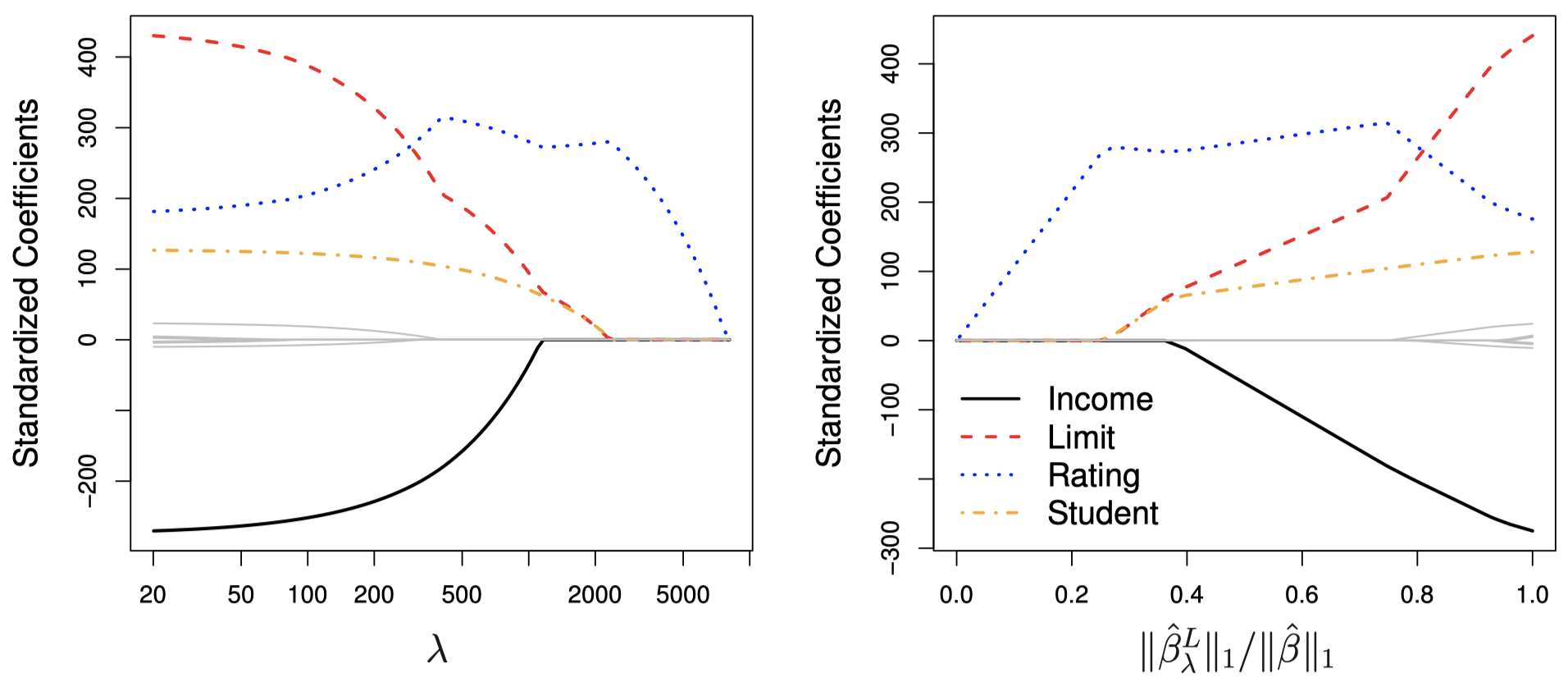### 3.3 岭回归和 Lasso 的其他形式

$\mathop{\operatorname{minimize}} \limits_{\beta} \sum_{i=1}^{n}\left(y_i - \beta_0 - \sum_{j=1}^{p}\beta_j x_{ij}\right)^2 \quad \text{subject to}\quad \sum_{j=1}^{p} |\beta_j| \le s$

$\mathop{\operatorname{minimize}} \limits_{\beta} \sum_{i=1}^{n}\left(y_i - \beta_0 - \sum_{j=1}^{p}\beta_j x_{ij}\right)^2 \quad \text{subject to}\quad \sum_{j=1}^{p} \beta_j^2 \le s$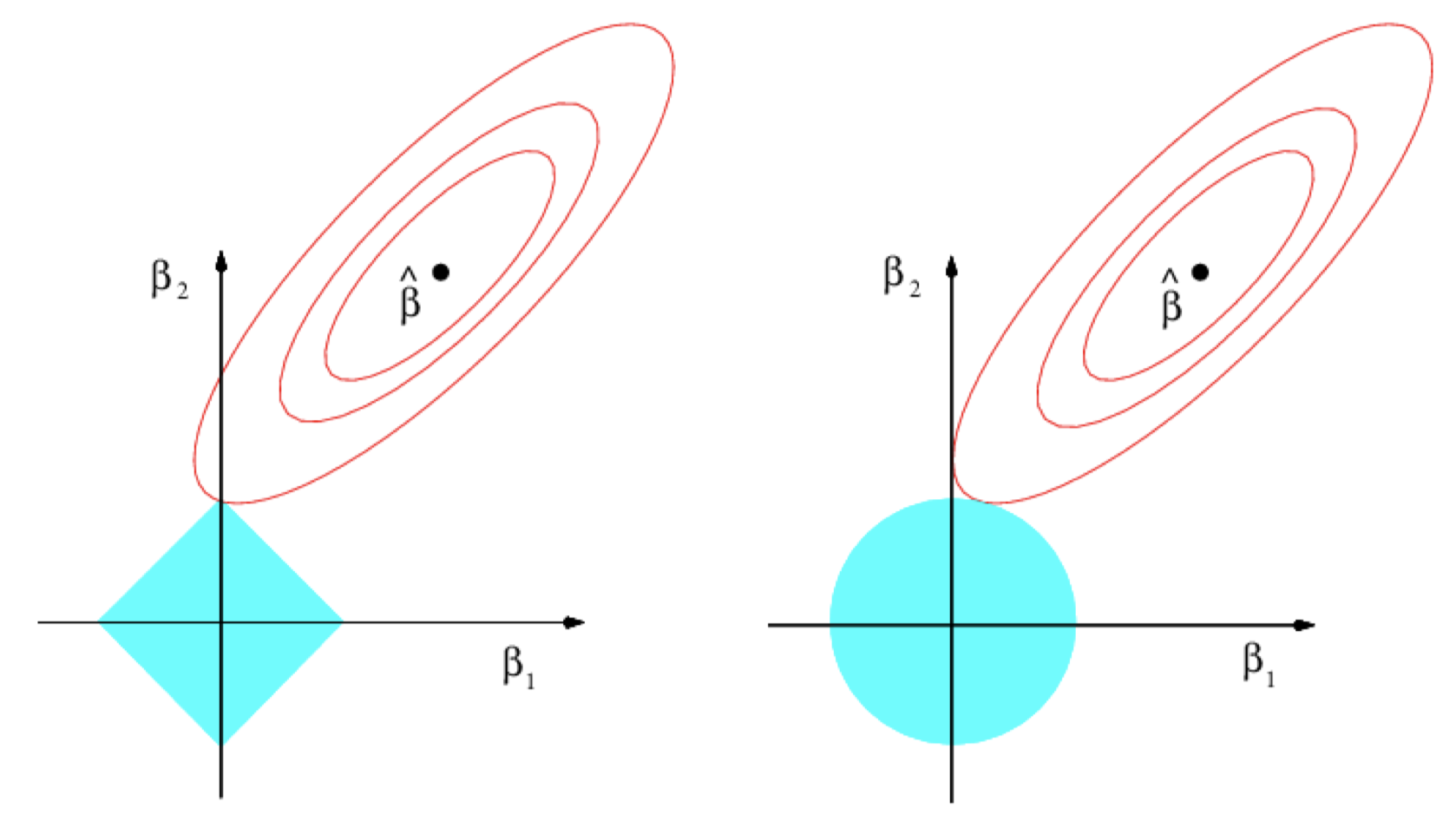### 3.4 对比 Lasso 和岭回归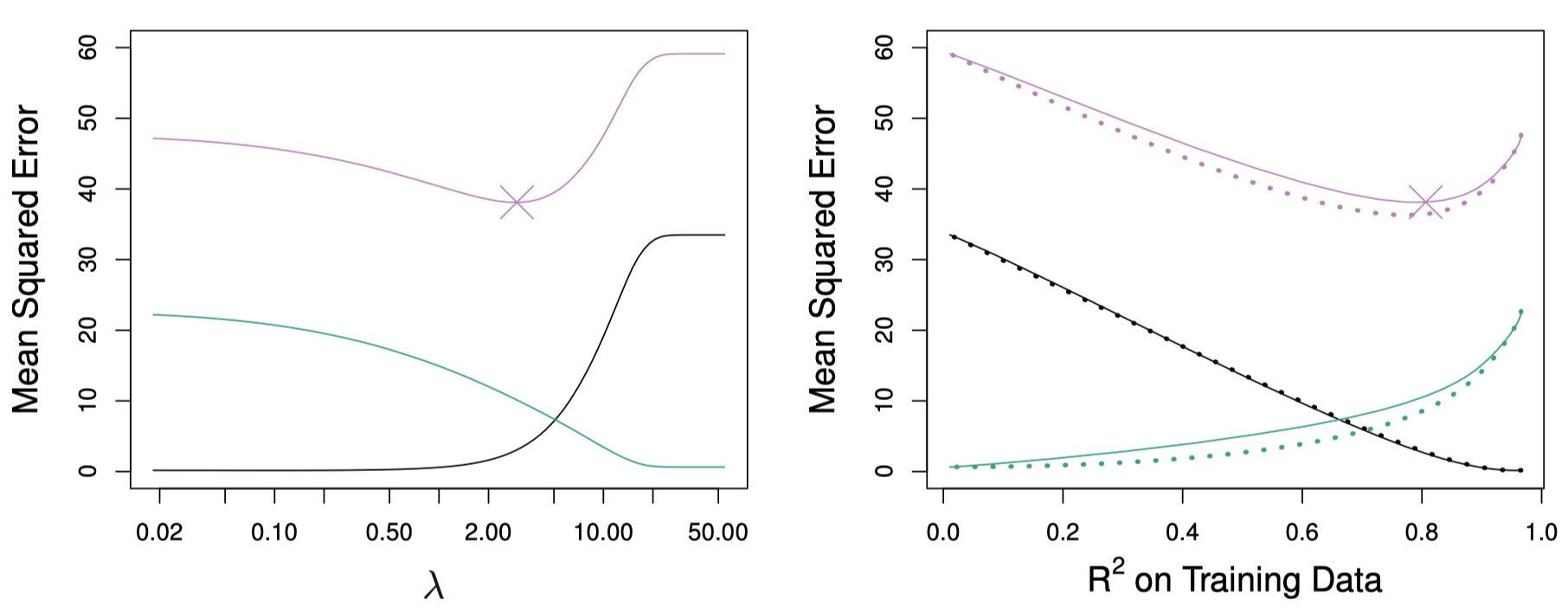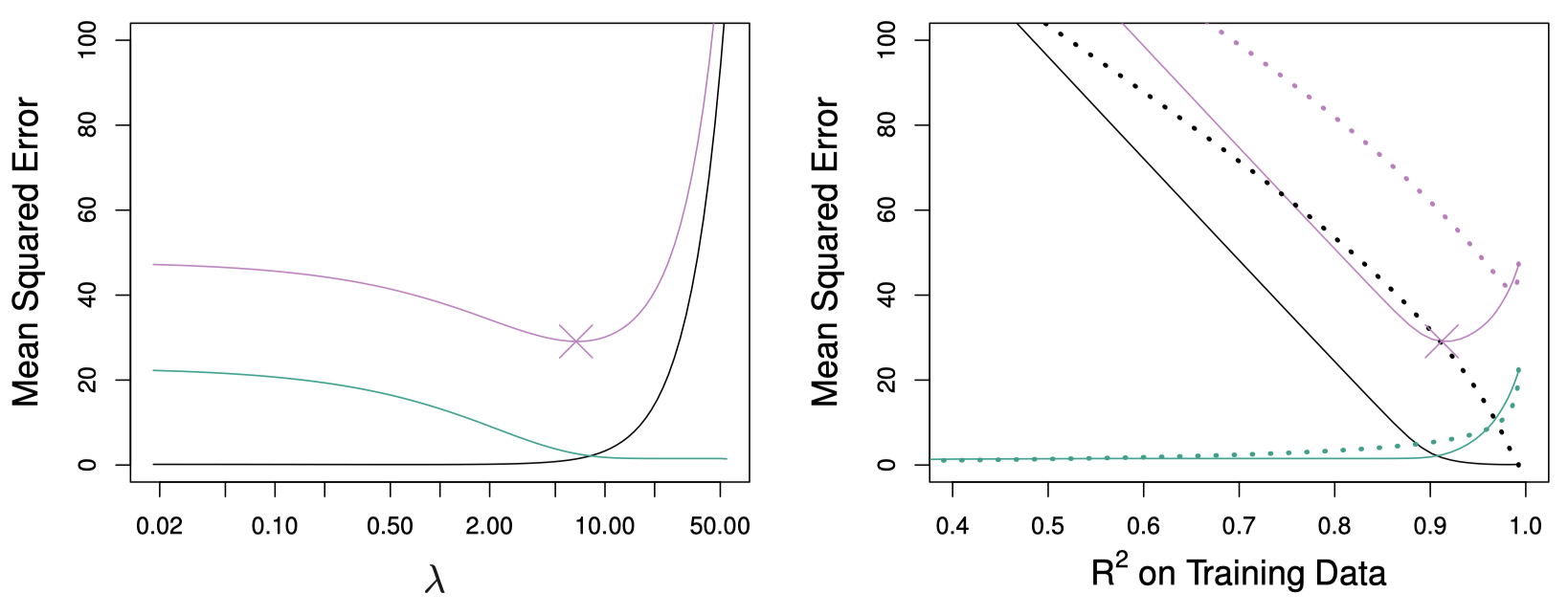### 3.5 选择岭回归和 Lasso 的调节参数

• 首先，选择一个由不同 $\lambda$ 值组成的网格，并且为其中的每个 $\lambda$ 值计算交叉验证错误率。

• 然后，选择交叉验证误差最小的调节参数值。

• 最后，使用所有可用的观测值和选定的调节参数值重新拟合模型。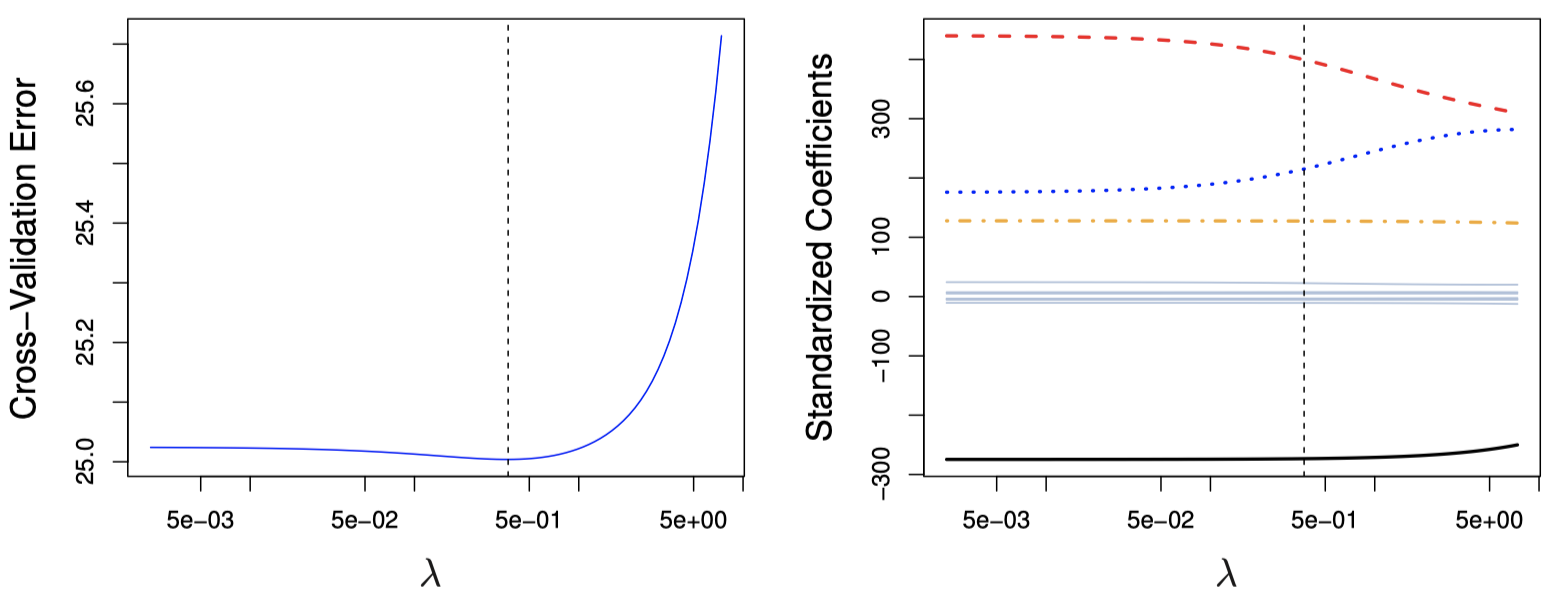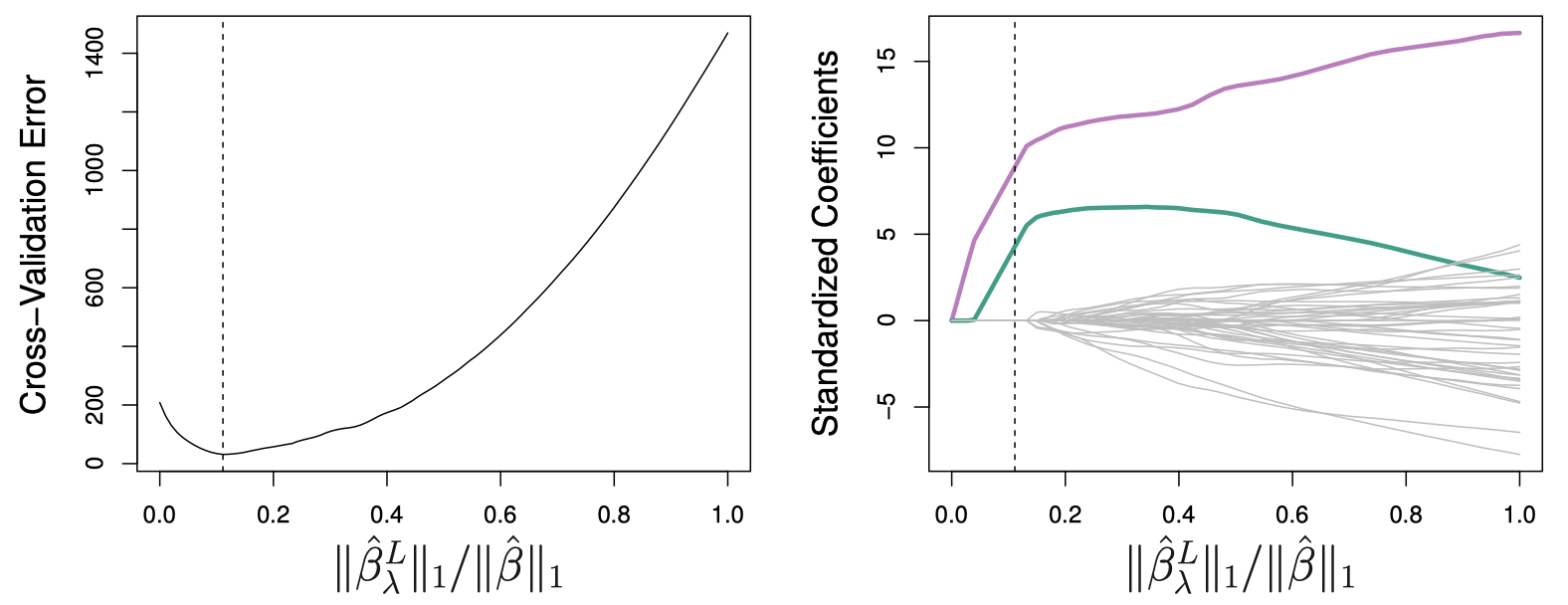## 4. 降维方法

$Z_m=\sum_{j=1}^{p}\phi_{jm}X_j$

$y_i = \theta_0 + \sum_{m=1}^{M}\theta_m z_{im} + \epsilon_i, \quad i=1,\dots,n$

$\sum_{m=1}^{M}\theta_m z_{im} = \sum_{m=1}^{M}\theta_m \sum_{j=1}^{p}\phi_{jm}x_{ij}= \sum_{j=1}^{p} \sum_{m=1}^{M} \theta_m \phi_{jm} x_{ij} = \sum_{j=1}^{p} \beta_j x_{ij}$

$\beta_j = \sum_{m=1}^{M} \theta_m \phi_{jm}$

### 4.1 主成分回归

• 第一个主成分是具有最大方差的 (经过归一化的) 变量线性组合。
• 第二个主成分是与第一主成分不相关的、具有最大方差的变量线性组合。
• 以此类推。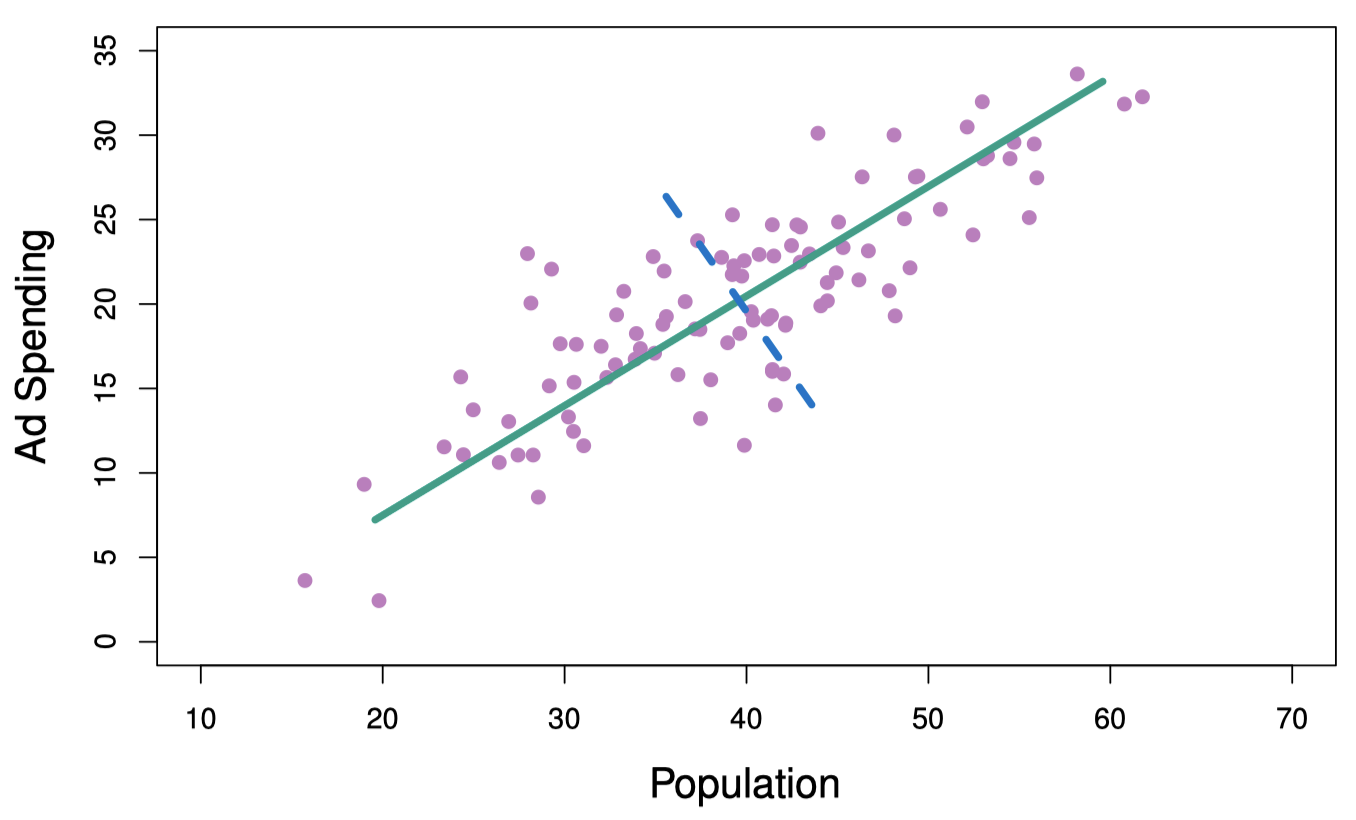$Z_1= 0.839 \times (\mathtt{pop} - \overline{\mathtt{pop}}) + 0.544 \times (\mathtt{ad} - \overline{\mathtt{ad}})$

$z_{i1} = 0.839 \times (\mathtt{pop}_i - \overline{\mathtt{pop}}) + 0.544 \times (\mathtt{ad}_i - \overline{\mathtt{ad}})$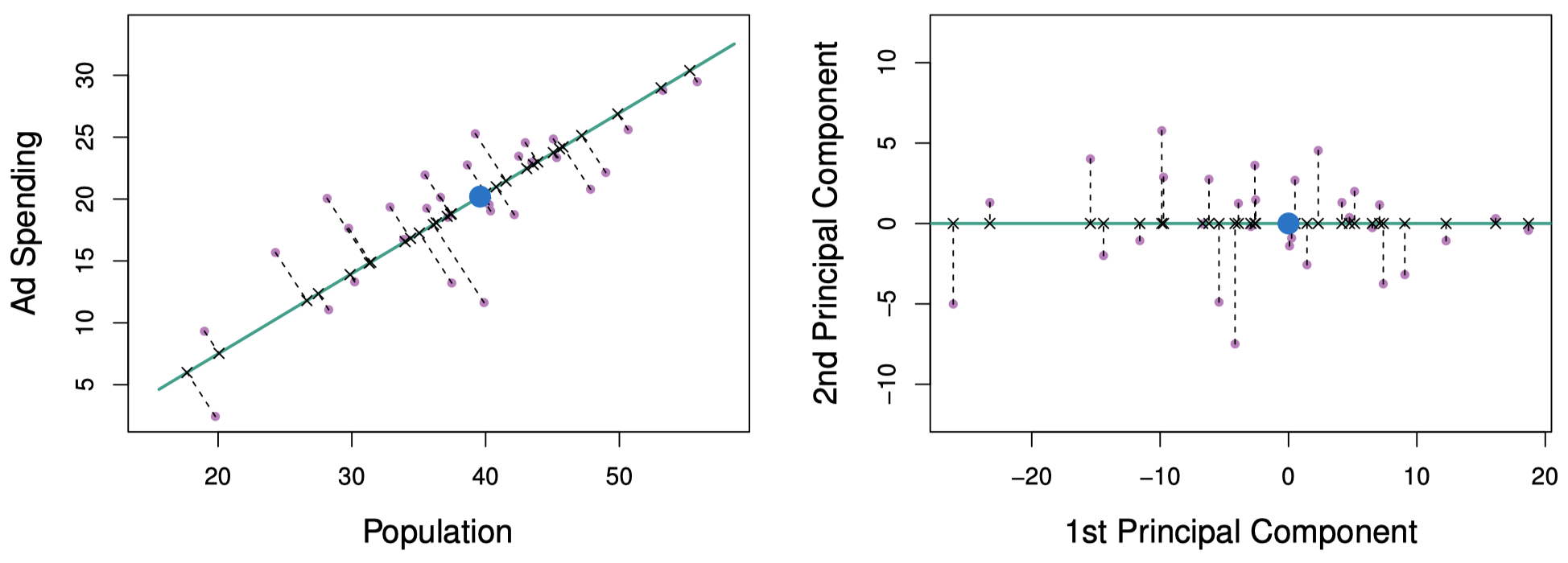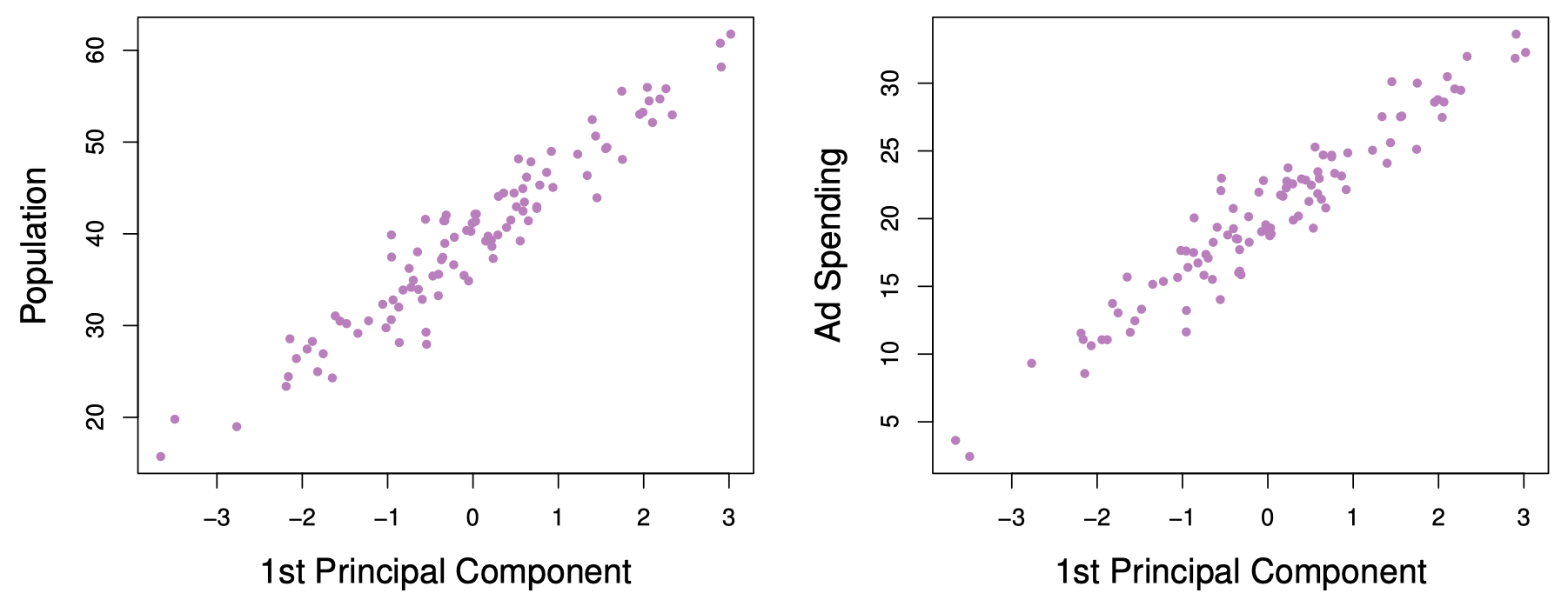$Z_2= 0.544 \times (\mathtt{pop} - \overline{\mathtt{pop}}) - 0.839 \times (\mathtt{ad} - \overline{\mathtt{ad}})$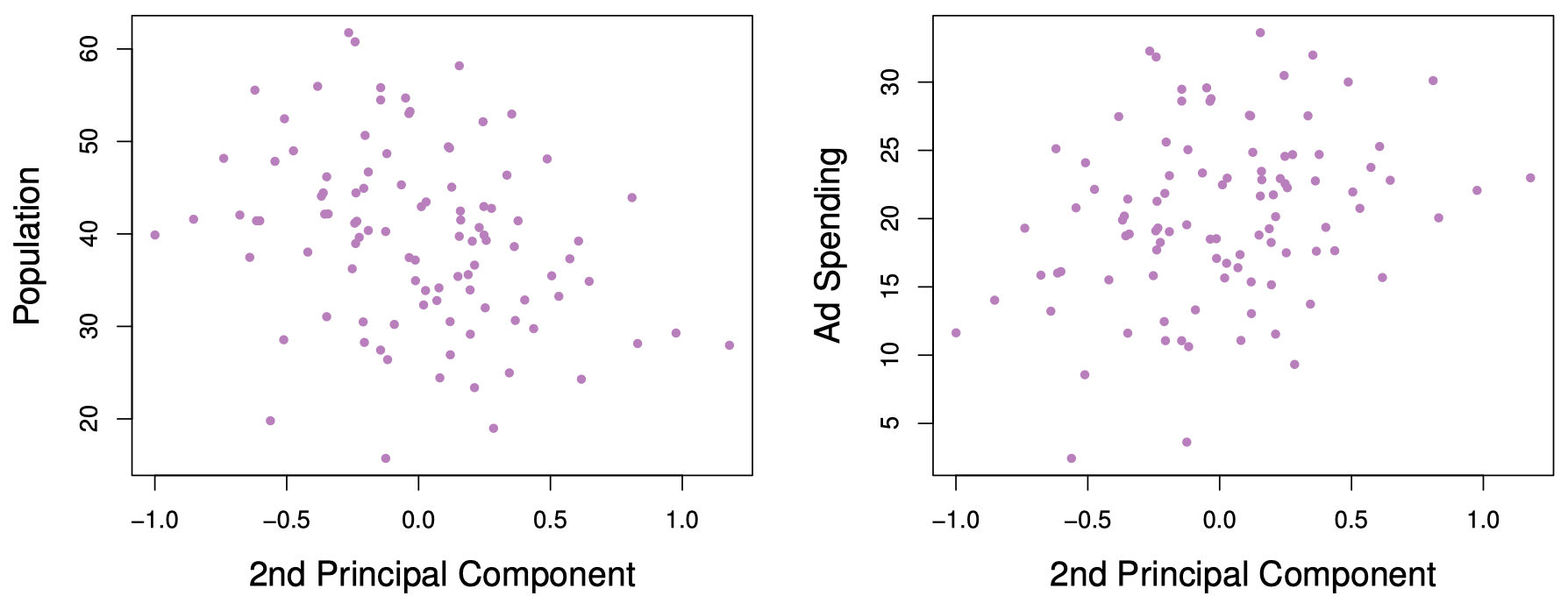#### 主成分回归的应用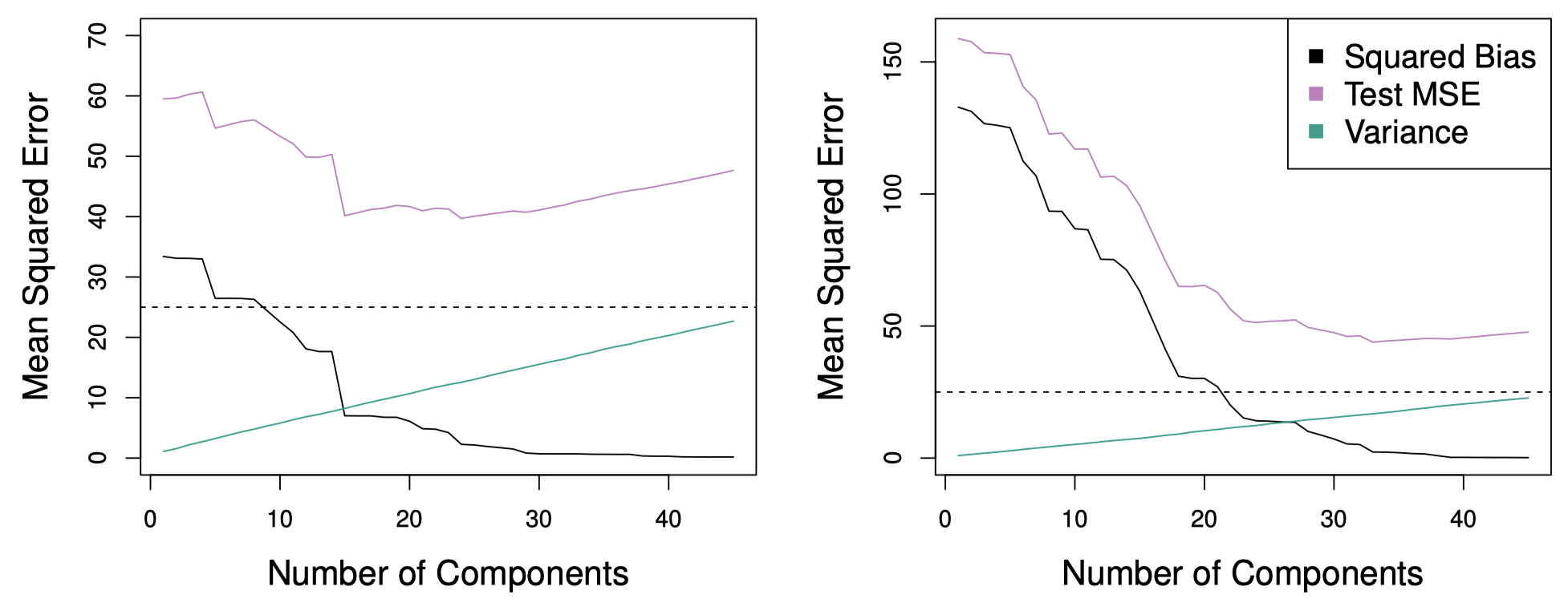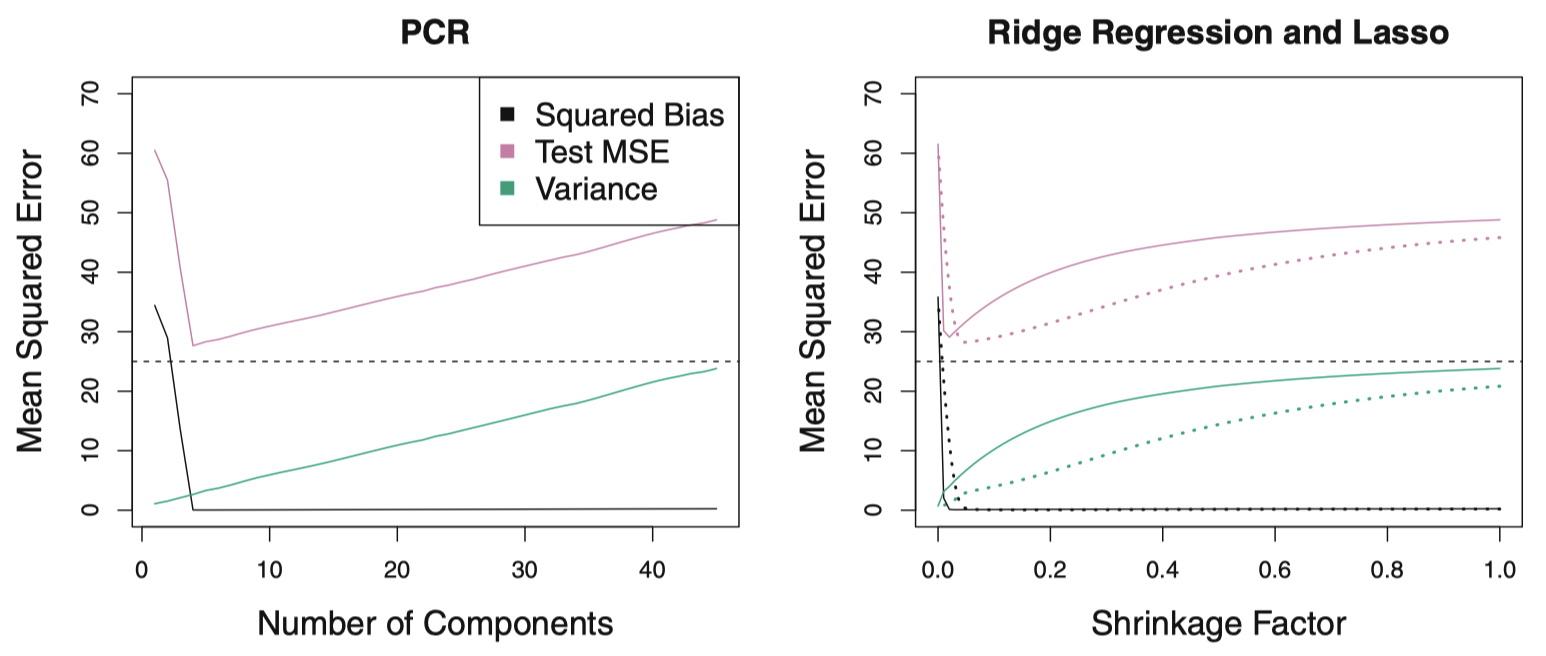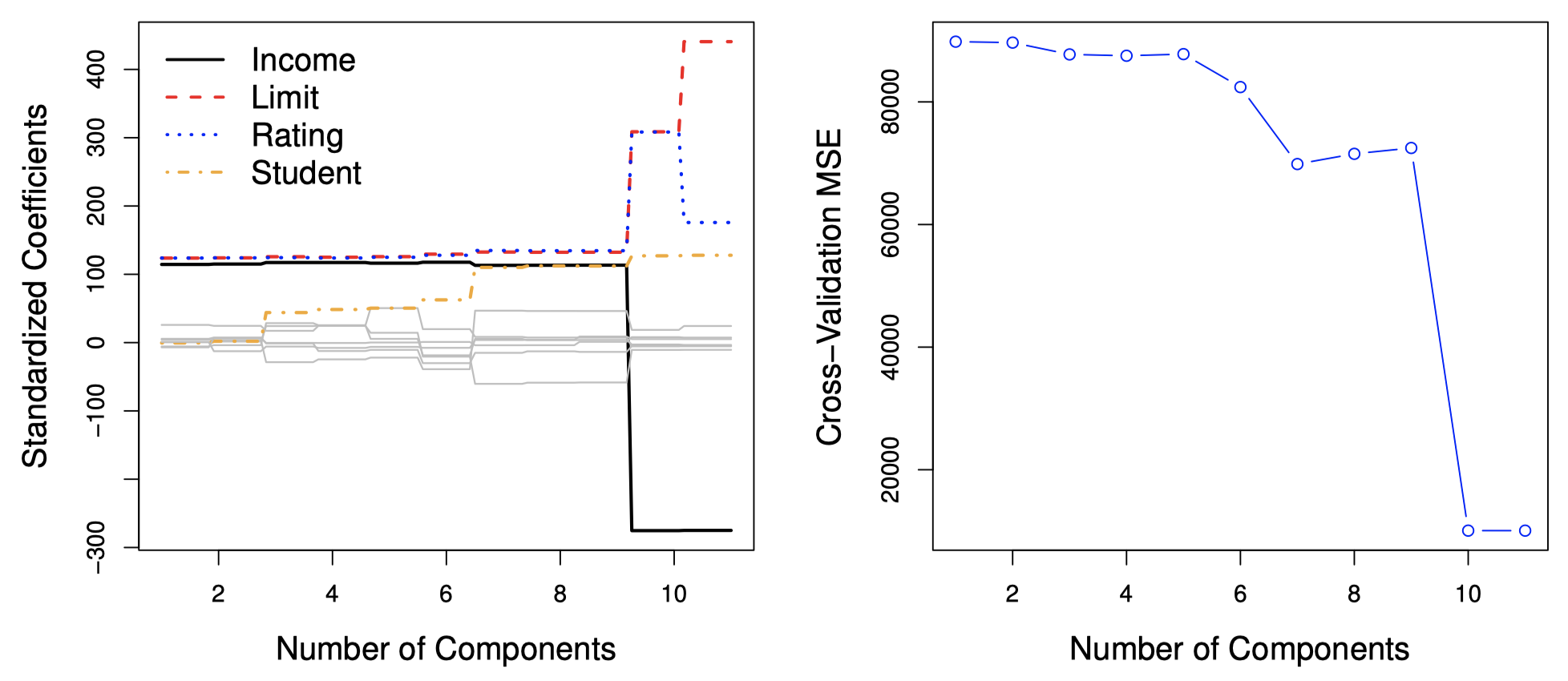### 4.2 偏最小二乘法

PCR 方法需要确定能够最大限度地代表预测变量 $X_1,\dots,X_p$ 的线性组合或方向。这些方向是通过 无监督 (unsupervised) 方法确定的，因为该过程中没有使用响应变量 $Y$ 来帮助确定主成分方向。也就是说，响应变量没有指导主成分的构造过程。因此，PCR 存在一个潜在的严重缺陷：我们无法保证那些能够很好地解释预测变量的方向同样可以很好地帮助预测响应变量。

PLS 在化学统计学领域应用广泛，许多变量是数字化的光谱数据。在实践中，其表现通常没有岭回归或 PCR 好。作为有监督降维技术，PLS 虽然可以减小偏差，但同时可能会增大方差，所以总体而言，PLS 与 PCR 各有优劣。

## 5. 总结

• 模型选择方法是进行数据分析的重要工具，尤其是对于涉及许多预测变量的大型数据集。

• 关于 稀疏 (sparsity) 方法 (例如：Lasso) 的研究是一个非常热门的领域。

• 在后面课程中，我们将更详细地介绍稀疏性，包括诸如 弹性网 (elastic net) 之类的相关方法。本作品采用知识共享署名-非商业性使用-相同方式共享 4.0 国际许可协议进行许可。 欢迎转载，并请注明来自：YEY 的博客 同时保持文章内容的完整和以上声明信息！